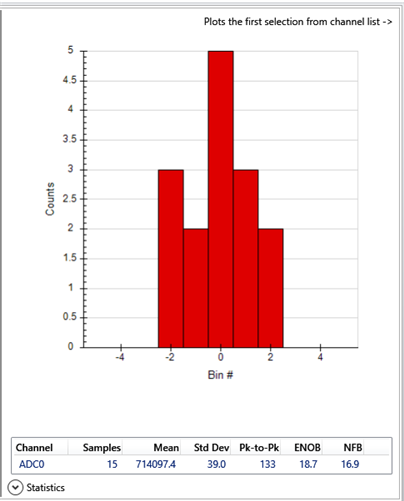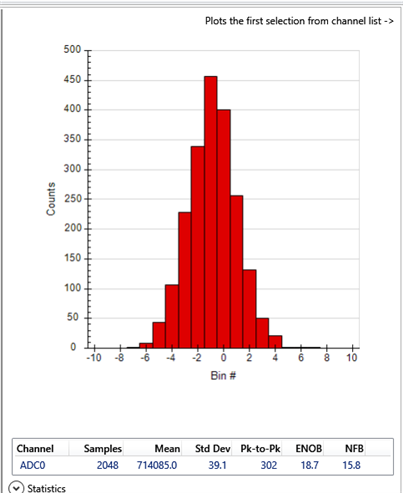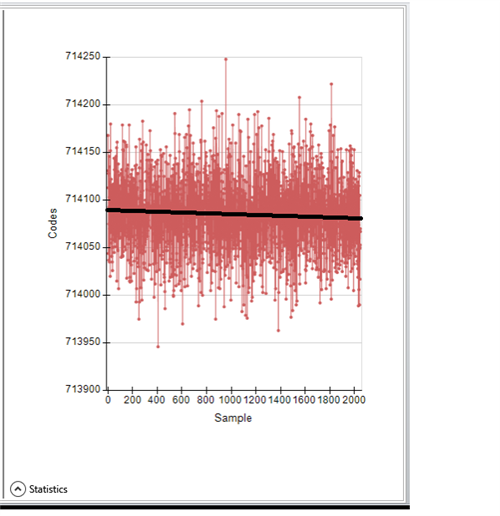If you have a related question, please click the "Ask a related question" button in the top right corner. The newly created question will be automatically linked to this question.

Hi,

I'm using a ADS122C04EVM kit for testing the ADS122C04 for RTD measurement. I had start with Delta-Sigma ADC EvaluaTIon SOftware. All is working properly but when i replace the RTD by a 100Ohms précision résistor i don't obtain a beter stability than +/-0.05°C. Is there some easy filter modifications someone can give me for having a better stability with this board? Or some other solution?

This results was obtained using the 3-wire RTD predefined script and Data Analysis "CollectData" default function .

Many thanks if someone can help me.

You will find attached an example of log file:

log 20220131-1.txt

• Hi Julien,

There are 2 main components to stability and that is noise and drift.  If we look strictly at the noise, the best the ADC is capable of doing is as given in the datasheet noise tables.  If we look at the numbers for a gain of 4 at 20sps we see a value of 5.74uV p2p (peak - 2 - peak).  However the table is created from a measurement that is made on a limited time period of 750ms.  This would equate to 15 samples at 20sps (50ms period) data output rate (750ms / 50ms = 15 samples).  Below is shown the first 15 samples from the dataset.Note that the p2p value is 133 codes.  For the ratiometric measurement being made the value of one code = +/- (4700 *250uA)/4/2^24 = 35nV.  If we calculate the p2p voltage we have 133 * 35nV = 4.65uV p2p which is actually slightly better that the noise table data of 5.74uV p2p.  So the noise performance from the ADC is quite good.

If we analyze the long term data of 2048 samples (2048 samples * 50ms period = 102.4 seconds) we see the following from the data you sent.If we look at the time domain plot we see the following:Here we see a drift component as well as the noise.  There can be drift from a number of sources.  As the measurement is ratiometric, any drift from the IDAC source will cancel in the measurement.  However drift of the ADC, reference resistor or input resistor will add error.

There are a couple of large noise excursions which account for most of the deviation over the time period.  The peaks occur at approximately sample 400 and 1000.  One large improvement would be to use a moving average, but unfortunately the EVM firmware does not allow it.

Best regards,

Bob B